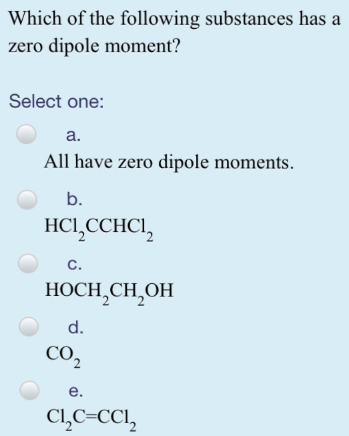# Problem: Which of the following substances hasa zero dipole moment? Select one: a. All have zero dipole moments b. HCl2CCHCl2 c. HOCH2CH2OH d. CO2e. CI2C=CCl2

###### FREE Expert Solution

If we look at the formula of the choices they all have pretty symmetric. What you halve on the first half is exactly what you have on the second half. This would be more apparent if you draw the lewis structures. CO2 is the one that is the least obvious but we know that C is the central atom here so still CO2 is symmetric.

92% (140 ratings)###### Problem Details

Which of the following substances hasa zero dipole moment?

Select one:

a. All have zero dipole moments

b. HCl2CCHCl2

c. HOCH2CH2OH

d. CO2

e. CI2C=CCl2